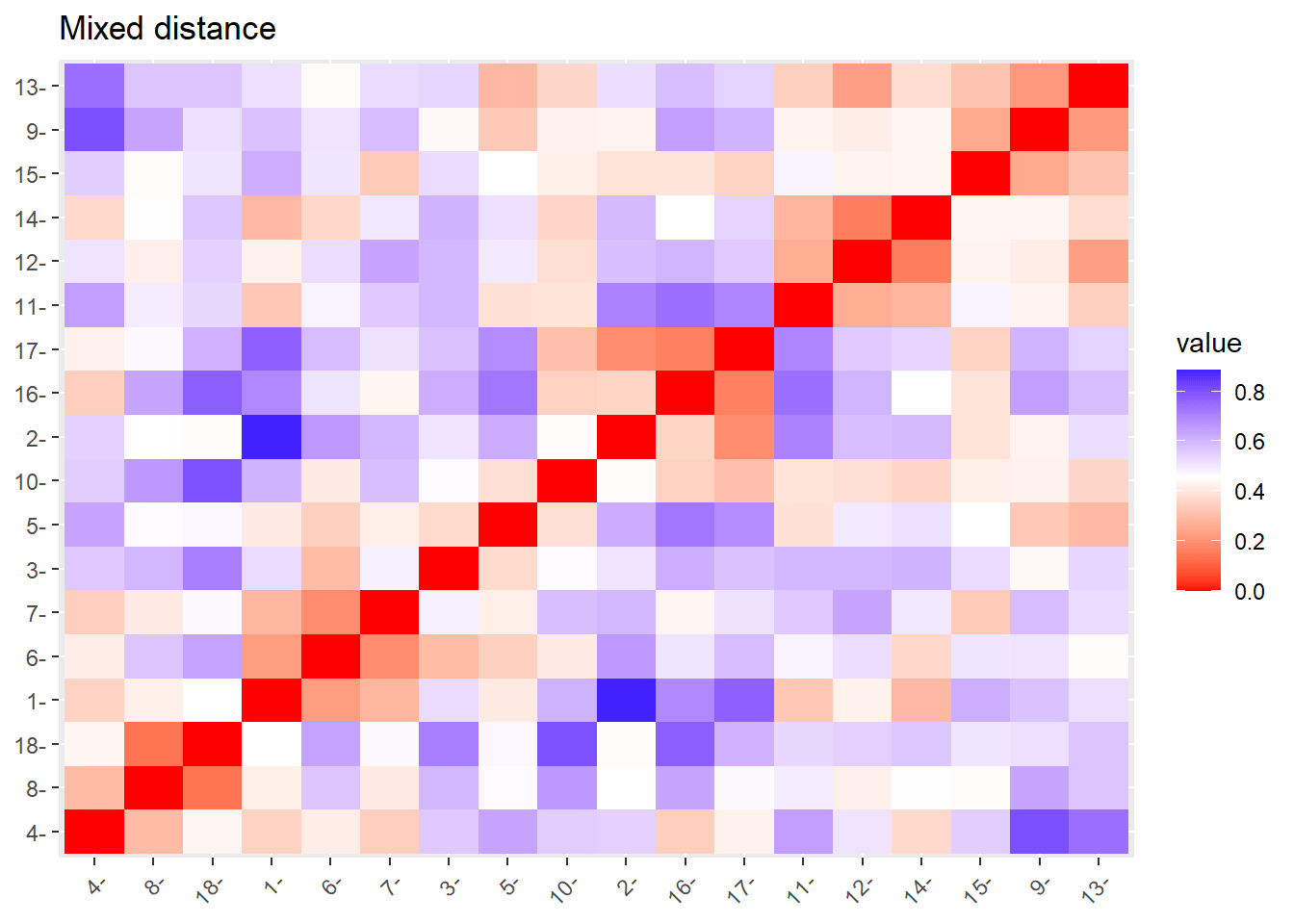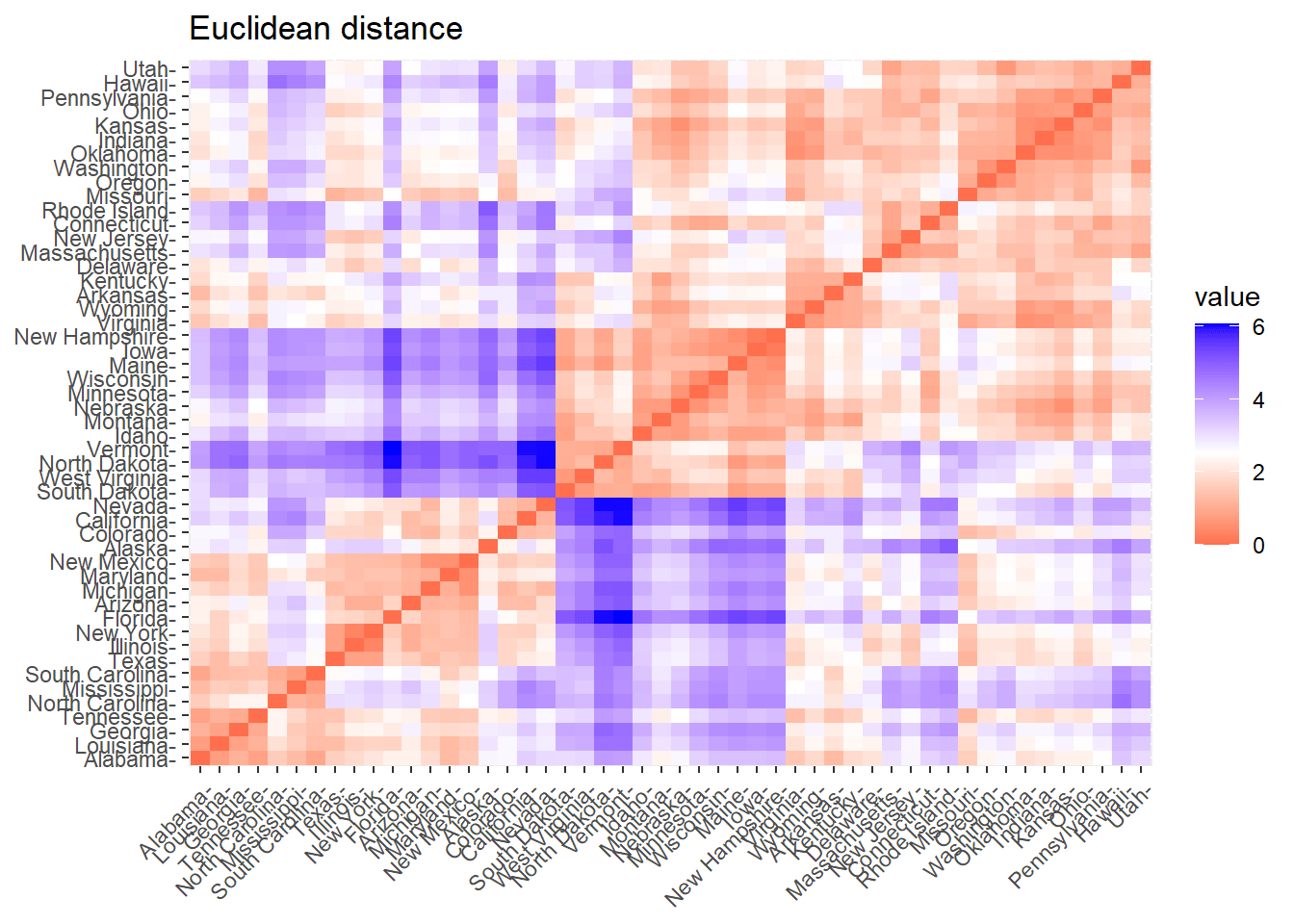[This article was first published on R tutorials – Statistical Aid: A School of Statistics, and kindly contributed to R-bloggers]. (You can report issue about the content on this page here)
Want to share your content on R-bloggers? click here if you have a blog, or here if you don't.

Cluster analysis is a statistical technique that groups similar observations into clusters based on their characteristics. It is a statistical method of processing data. A good cluster analysis produces high-quality clusters with high inter-class correlation. This blogpost contains the following steps of cluster analysis:

• Introduction
• Packages used
• Import data file
• Handling with missing values
• Scaling of the data
• Distance matrix computation
• Vidualising distances

## Introduction to cluster analysis

Clustering is the task of dividing the population or data points into a number of groups such that data points in the same groups are more similar to other data points in the same group and dissimilar to the data points in other groups. It is one of the important data mining methods for discovering knowledge in multidimensional data. The goal of clustering is to identify patterns or groups of similar objects within a data set of interest.

## Packages used in cluster analysis

There are several packages used for cluster analysis. I shall use two packages named as `cluster` and `factoextra` packages. You can install these packages by using `install.packages()` function. This will take some time while installing package files from cran. Cluster package is used for distance measures while `factoextra` package is used for ggplot2 based elegant visualization of clustering results.

```install.packages("cluster")
install.packages("factoextra")
# OR install both packages with single command
install.packages(c("cluster", "factoextra"))```

To load or attach these packages use `library()` or `require()` functions. Both these functions load the namespace of the package with the named package and attach it to the search list. Both functions check and update the list of currently attached packages and do not reload a namespace that is already loaded.

```Use .packages(all = TRUE) to obtain just the names of all available packages
library("cluster")
library("factoextra")```

### Import data file

Before importing data in R first thing to do is to prepare the data file according to the given instructions.

• Use first row as `column names` that represent variables
• Use first column as `row names` that represent observations
• Avoid column names with `blank spaces`. For example, good name for plant height is to place underscore or dot between the two words e.g `plant_height` or `plant.height`. A bad name for plant height is to give space between the two words (plant height).
• Avoid name with `special symbols`.
• Avoid beginning variable names with a number. Use letter instead e.g instead of 1000_grain_weight type `th_grain_weight` or `grain_weight_1000`.
• Avoid `blank rows` in your data
• Delete any `comments` in your file
• Replace `missing values` with NA using `na.omit()` function
• Use four digit format for column containing date

After preparing the file, next step is to save the file. Save the file either as `.CSV` format. There are several built-in demo data sets in R for playing with R functions. These include `USArrests``iris` and `mtcars`. To load a demo data set you can use the `data()` function. In this example `USArrests` data set will be used to perform cluster analysis in R. Using `head()` function will print the first six rows of the data set.

```data = USArrests
#            Murder Assault UrbanPop Rape
# Alabama      13.2     236       58 21.2
# Alaska       10.0     263       48 44.5
# Arizona       8.1     294       80 31.0
# Arkansas      8.8     190       50 19.5
# California    9.0     276       91 40.6
# Colorado      7.9     204       78 38.7```

### Handling with missing values in cluster analysis

To see whether the data contain missing values or not, use `na.fail()` function which returns the object if it does not contain any missing values. If data have missing values then use `na.omit()` function to remove the incomplete cases.

```na.pas() function returns the object unchanged
data = na.omit(data)```

### Scaling of the data

Scaling of the data is carried out as we do not want clustering to depend on an arbitrary value. This is particularly recommended when variables are measured in different scales. The scaling goal is to make variables more comparable.

`Generally variables are scaled to have one value for standard deviation and zero value for mean. `

The data is also standardized if the mean and standard deviation of variables is largely different. Scaling will transform the data as the ratio between two deviations with numerator as mean deviation or median deviation and denominator as standard deviation or interquartile range or median absolute range.

This approach is widely used in gene expression data analysis before clustering. Use `scale()` function in the R console to standardize the data.

```data.scaled <- scale(data)
#                Murder   Assault   UrbanPop         Rape
# Alabama    1.24256408 0.7828393 -0.5209066 -0.003416473
# Alaska     0.50786248 1.1068225 -1.2117642  2.484202941
# Arizona    0.07163341 1.4788032  0.9989801  1.042878388
# Arkansas   0.23234938 0.2308680 -1.0735927 -0.184916602
# California 0.27826823 1.2628144  1.7589234  2.067820292
# Colorado   0.02571456 0.3988593  0.8608085  1.864967207```

### Distance matrix computation

For computing distance measures we can use three methods which include;

• Euclidean distance
• correlation based distance
• distance for mixed data

#### Euclidean distance

First, we shall see how to compute Euclidean distance. The function `dist()` from stats package will be used to compute a specified distance measures to compute the distance between the rows of a data matrix. Specify a numeric matrix, data frame or dist object in `x` argument. In `method` argument you can type the distance measure to be used.

`method`: “euclidean”, “maximum”, “manhattan”, “canberra”, “binary” or “minkowski”

To make it easier to see the distance information generated by `dist()` function, round the values in the distance vector using `as.matrix()` function. Here the range of 1 to 3 represents the first three columns and the first three rows, respectively. The value 1 represents output will be round to one decimal place.

```data.eucl = dist(x = data.scaled,
method = "euclidean")
round(as.matrix(data.eucl)[1:3, 1:3], 1)
# Alabama     0.0    2.7     2.3
# Arizona     2.3    2.7     0.0```

In this distance matrix result, the values represent the distance between the objects. The values in the diagonal represent the distance between the objects and themselves which are zero.

#### Correlation based distance

The second type is to compute correlation-based distance measures. This type is commonly used in gene expression data analysis. To compute correlation-based distances use `get_dist()` function after loading `factoextra` package by using library function.

`method`: “Pearson”, “Spearman” or “Kendall”

`Pearson` correlation is the most commonly used method. It is also known as a parametric correlation which depends on the distribution of the data. `Kendall` and `Spearman` correlations are non-parametric associations that are used to perform rank-based correlation analysis.

```library(factoextra)
data.cor <- get_dist(x = data.scaled,
method = "pearson")
round(as.matrix(data.cor)[1:3, 1:3], 1)
# Alabama     0.0    0.7     1.4
# Arizona     1.4    0.8     0.0```

#### Distances for mixed data

The above two distance measuring methods accepts numeric data. However, for data containing both numeric and non-numeric or mixed data, `daisy()` function is used to compute the distances. In daisy function, the Gower’s coefficient which is one of the most popular measures of proximity for mixed data types will be used as the metric.

Here we shall use a different example of flower data which contain factor, ordered factor and numeric variables. For this purpose first load `cluster` package using `library()` function. Then load the R demo data set `flower` using the `data()` function.

```library(cluster)
data(flower)
str(flower)
# 'data.frame': 18 obs. of  8 variables:
#  \$ V1: Factor w/ 2 levels "0","1": 1 2 1 1 1 1 1 1 2 2 ...
#  \$ V2: Factor w/ 2 levels "0","1": 2 1 2 1 2 2 1 1 2 2 ...
#  \$ V3: Factor w/ 2 levels "0","1": 2 1 1 2 1 1 1 2 1 1 ...
#  \$ V4: Factor w/ 5 levels "1","2","3","4",..: 4 2 3 4 5 4 4 2 3 5 ...
#  \$ V5: Ord.factor w/ 3 levels "1"<"2"<"3": 3 1 3 2 2 3 3 2 1 2 ...
#  \$ V6: Ord.factor w/ 18 levels "1"<"2"<"3"<"4"<..: 15 3 1 16 2 12 13 7 4 14 ...
#  \$ V7: num  25 150 150 125 20 50 40 100 25 100 ...
#  \$ V8: num  15 50 50 50 15 40 20 15 15 60 ...```

To compute the distance for mixed variables use `daisy()` function. Round the distance matrix to two decimal place using `as.matrix()` function.

```data.daisy <- daisy(flower)
round(as.matrix(data.daisy)[1:3, 1:3], 2)
#      1    2    3
# 1 0.00 0.89 0.53
# 2 0.89 0.00 0.51
# 3 0.53 0.51 0.00```

### Visualizing distances

After measuring distances next you need to visualize the distance matrix. A simple way to visualize distance matrices is to use the `fviz_dist()` function by first loading the `factoextra` package.

```This function classify data samples into groups of similar objects.
library(factoextra)
# Visualize Euclidean matrix
fviz_dist(dist.obj = data.eucl,
order = TRUE, show_labels = TRUE)
# Visualize correlation matrix
fviz_dist(dist.obj = data.cor,
order = TRUE, show_labels = TRUE)
# Visualize mixed data distance matrix
fviz_dist(dist.obj = data.daisy,
order = TRUE, show_labels = TRUE)```

The red color indicates high similarity while blue color indicates low similarity. The color level is proportional to the value of dissimilarity between observations where pure red represents zero and pure blue represents one.The post Cluster analysis using R appeared first on Statistical Aid: A School of Statistics.